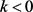﻿ N-Cell模糊数上的近似大于等于关系

# N-Cell模糊数上的近似大于等于关系Approximately Greater Than or Equal Relation on Fuzzy N-Cell Numbers

Abstract: In this paper, we introduce the approximately greater than or equal relation on fuzzy n-cell num-bers and investigate its properties. We focus on the variation of this relationship under addition, subtraction, multiplication, division and scalar-multiplication.

1. 引言

Buckley   率先提出了一维模糊数近似大于、近似等于以及近似大于等于关系的概念，并将其应用于具有模糊性决策对象的排序中。吴望名在  中提出了一维模糊数相容的概念。王绪柱在  中针对Buckley在文  中提出的近似相等关系给出了易于判定的充要条件，并讨论了一维模糊数近似相等的性质。但是，在实际应用中，一维模糊数往往无法满足需求。因此，王桂祥、吴从炘在  中提出了n-cell模糊数的概念，n-cell模糊数是一种特殊的n维模糊数，同时也是对一维模糊数的推广。王桂祥及其团队   将n-cell模糊数应用于分类、模式识别、信息融合等领域中，受到了广泛的关注，因此，讨论n-cell模糊数上的近似大于等于关系也是非常重要的。

2. 预备知识

$u:{R}^{n}\to \left[0,1\right]$ 满足以下性质(1)-(4)：

1) u是正规的模糊集，即存在 ${x}_{0}\in {R}^{n}$ 使得 $u\left({x}_{0}\right)=1$

2) u是凸模糊集，即对任意 $x,y\in {R}^{n},\lambda \in \left[0,1\right]$$u\left(\lambda x+\left(1-\lambda \right)y\right)\ge \mathrm{min}\left\{u\left(x\right),u\left(y\right)\right\}$

3) u是上半连续函数，即 $\underset{x\to {x}_{0}}{\mathrm{lim}\mathrm{sup}}u\left(x\right)\le u\left({x}_{0}\right)$

4) ${\left[u\right]}_{0}$ 有界。

$a$ 表示 ${R}^{n}$ 中的点 $\left(a,a,\cdots ,a\right)$ ，用表示模糊集 $\stackrel{^}{a}:{R}^{n}\to \left[0,1\right]$$\stackrel{^}{a}\left(x\right)=\left\{\begin{array}{l}0,x\ne a,\\ 1,x=a.\end{array}$ 在不引起混淆的情况下，我们也用 $a$ 表示 $\stackrel{^}{a}$ ，此时对任意的 $\alpha \in \left[0,1\right]$${\left[a\right]}_{\alpha }=\left\{a\right\}$

$u\in L\left({E}^{n}\right)$ ，若 ${u}_{i}^{-}\left(0\right)>0,i=1,2,\cdots ,n$ ，则称u为正n-cell模糊数；若 ${u}_{i}^{+}\left(0\right)<0$$i=1,2,\cdots ,n$ ，则称u为负n-cell模糊数。

N-cell模糊数的代数运算如下：对任意 $u,v\in L\left({E}^{n}\right),k\in R,\alpha \in \left[0,1\right]$ ，有：

${\left[u+v\right]}_{\alpha }={\left[u\right]}_{\alpha }+{\left[v\right]}_{\alpha }=\underset{i=1}{\overset{n}{\prod }}\left[{u}_{i}^{-}\left(\alpha \right)+{v}_{i}^{-}\left(\alpha \right),{u}_{i}^{+}\left(\alpha \right)+{v}_{i}^{+}\left(\alpha \right)\right],$

${\left[u-v\right]}_{\alpha }={\left[u\right]}_{\alpha }-{\left[v\right]}_{\alpha }=\underset{i=1}{\overset{n}{\prod }}\left[{u}_{i}^{-}\left(\alpha \right)-{v}_{i}^{+}\left(\alpha \right),{u}_{i}^{+}\left(\alpha \right)-{v}_{i}^{-}\left(\alpha \right)\right],$

${\left[ku\right]}_{\alpha }=k{\left[u\right]}_{\alpha }=\left\{\begin{array}{l}\underset{i=1}{\overset{n}{\prod }}\left[k{u}_{i}^{-}\left(\alpha \right),k{u}_{i}^{+}\left(\alpha \right)\right],k\ge 0,\\ \underset{i=1}{\overset{n}{\prod }}\left[k{u}_{i}^{+}\left(\alpha \right),k{u}_{i}^{-}\left(\alpha \right)\right],k\le 0,\end{array}$

$\begin{array}{l}{\left[uv\right]}_{\alpha }=\underset{i=1}{\overset{n}{\prod }}\left[\mathrm{min}\left\{{u}_{i}^{-}\left(\alpha \right){v}_{i}^{-}\left(\alpha \right),{u}_{i}^{-}\left(\alpha \right){v}_{i}^{+}\left(\alpha \right),{u}_{i}^{+}\left(\alpha \right){v}_{i}^{-}\left(\alpha \right),{u}_{i}^{+}\left(\alpha \right){v}_{i}^{+}\left(\alpha \right)\right\},\\ \text{\hspace{0.17em}}\text{\hspace{0.17em}}\text{\hspace{0.17em}}\text{\hspace{0.17em}}\text{\hspace{0.17em}}\text{\hspace{0.17em}}\text{\hspace{0.17em}}\text{\hspace{0.17em}}\text{\hspace{0.17em}}\text{\hspace{0.17em}}\text{\hspace{0.17em}}\text{\hspace{0.17em}}\text{\hspace{0.17em}}\text{\hspace{0.17em}}\text{\hspace{0.17em}}\text{\hspace{0.17em}}\mathrm{max}\left\{{u}_{i}^{-}\left(\alpha \right){v}_{i}^{-}\left(\alpha \right),{u}_{i}^{-}\left(\alpha \right){v}_{i}^{+}\left(\alpha \right),{u}_{i}^{+}\left(\alpha \right){v}_{i}^{-}\left(\alpha \right),{u}_{i}^{+}\left(\alpha \right){v}_{i}^{+}\left(\alpha \right)\right\}\right],\end{array}$

${\left[\frac{u}{v}\right]}_{\alpha }=\left[\mathrm{min}\left\{\frac{{u}_{i}^{-}\left(\alpha \right)}{{v}_{i}^{-}\left(\alpha \right)},\frac{{u}_{i}^{-}\left(\alpha \right)}{{v}_{i}^{+}\left(\alpha \right)},\frac{{u}_{i}^{+}\left(\alpha \right)}{{v}_{i}^{-}\left(\alpha \right)},\frac{{u}_{i}^{+}\left(\alpha \right)}{{v}_{i}^{+}\left(\alpha \right)}\right\},\mathrm{max}\left\{\frac{{u}_{i}^{-}\left(\alpha \right)}{{v}_{i}^{-}\left(\alpha \right)},\frac{{u}_{i}^{-}\left(\alpha \right)}{{v}_{i}^{+}\left(\alpha \right)},\frac{{u}_{i}^{+}\left(\alpha \right)}{{v}_{i}^{-}\left(\alpha \right)},\frac{{u}_{i}^{+}\left(\alpha \right)}{{v}_{i}^{+}\left(\alpha \right)}\right\}\right]$ (其中 $v$ 为正n-cell模糊数或负n-cell模糊数)。

Buckley在  中给出了一维模糊数近似大于等于关系的概念：

1) 若 $\nu \left(u\ge v\right)=1$$\nu \left(v\ge u\right)<\alpha$ ，则称 $u$$\alpha$ 水平下近似大于 $v$ ，记为 $u{\succ }_{\alpha }v$$v{\prec }_{\alpha }u$

2) 若 $\mathrm{min}\left(\nu \left(u\ge v\right),\nu \left(v\ge u\right)\right)\ge \alpha$ ，则称 $u$$v$$\alpha$ 水平下近似相等，记为 $u{\approx }_{\alpha }v$

3) 若 $u{\succ }_{\alpha }v$$u{\approx }_{\alpha }v$ ，则称 $u$$\alpha$ 水平下近似大于等于 $v$ ，记为 $u{\ge }_{\alpha }v$$v{\le }_{\alpha }u$

3. N-Cell模糊数上近似大于等于关系的性质

${\left[u-v\right]}_{\alpha }=\underset{i=1}{\overset{n}{\prod }}\left[{u}_{i}^{-}\left(\alpha \right)-{v}_{i}^{+}\left(\alpha \right),{u}_{i}^{+}\left(\alpha \right)-{v}_{i}^{-}\left(\alpha \right)\right]$

$u{\ge }_{\alpha }v$ 知： ${u}_{i}^{+}\left(\alpha \right)\ge {v}_{i}^{-}\left(\alpha \right),i=1,2,\cdots ,n$ 。则

${\left(u-v\right)}_{i}^{+}\left(\alpha \right)={u}_{i}^{+}\left(\alpha \right)-{v}_{i}^{-}\left(\alpha \right)\ge 0={0}_{i}^{-}\left(\alpha \right),i=1,2,\cdots ,n$

1) 若 $v$ 为正，则 $\frac{u}{v}{\ge }_{a}1$

2) 若 $v$ 为负，则 $\frac{u}{v}{\le }_{a}1$

${\left[\frac{u}{v}\right]}_{\alpha }=\underset{i=1}{\overset{n}{\prod }}\left[\mathrm{min}\left\{\frac{{u}_{i}^{-}\left(\alpha \right)}{{v}_{i}^{-}\left(\alpha \right)},\frac{{u}_{i}^{-}\left(\alpha \right)}{{v}_{i}^{+}\left(\alpha \right)}\right\},\mathrm{max}\left\{\frac{{u}_{i}^{+}\left(\alpha \right)}{{v}_{i}^{-}\left(\alpha \right)},\frac{{u}_{i}^{+}\left(\alpha \right)}{{v}_{i}^{+}\left(\alpha \right)}\right\}\right]$

$u{\ge }_{\alpha }v$ 知： ${u}_{i}^{+}\left(\alpha \right)\ge {v}_{i}^{-}\left(\alpha \right),i=1,2,\cdots ,n$ 。则

$\frac{{u}_{i}^{+}\left(\alpha \right)}{{v}_{i}^{-}\left(\alpha \right)}\ge 1={1}_{i}^{-}\left(\alpha \right),i=1,2,\cdots ,n$

${\left(\frac{u}{v}\right)}_{i}^{+}\left(\alpha \right)=\mathrm{max}\left\{\frac{{u}_{i}^{+}\left(\alpha \right)}{{v}_{i}^{-}\left(\alpha \right)},\frac{{u}_{i}^{+}\left(\alpha \right)}{{v}_{i}^{+}\left(\alpha \right)}\right\}\ge \frac{{u}_{i}^{+}\left(\alpha \right)}{{v}_{i}^{-}\left(\alpha \right)}\ge {1}_{i}^{-}\left(\alpha \right),i=1,2,\cdots ,n$

(2)证明与(1)类似。

1) $u+\omega {\ge }_{\alpha }v+\omega$

2) $u-\omega {\ge }_{\alpha }v-\omega$

${\left[u+\omega \right]}_{\alpha }=\underset{i=1}{\overset{n}{\prod }}\left[{u}_{i}^{-}\left(\alpha \right)+{\omega }_{i}^{-}\left(\alpha \right),{u}_{i}^{+}\left(\alpha \right)+{\omega }_{i}^{+}\left(\alpha \right)\right]$

${\left[v+\omega \right]}_{\alpha }=\underset{i=1}{\overset{n}{\prod }}\left[{v}_{i}^{-}\left(\alpha \right)+{\omega }_{i}^{-}\left(\alpha \right),{v}_{i}^{+}\left(\alpha \right)+{\omega }_{i}^{+}\left(\alpha \right)\right]$

$u{\ge }_{\alpha }v$ 知： ${u}_{i}^{+}\left(\alpha \right)\ge {v}_{i}^{-}\left(\alpha \right),i=1,2,\cdots ,n$ 。则

${u}_{i}^{+}\left(\alpha \right)+{\omega }_{i}^{+}\left(\alpha \right)\ge {v}_{i}^{-}\left(\alpha \right)+{\omega }_{i}^{+}\left(\alpha \right)\ge {v}_{i}^{-}\left(\alpha \right)+{\omega }_{i}^{-}\left(\alpha \right),i=1,2,\cdots ,n$

${\left(u+\omega \right)}_{i}^{+}\left(\alpha \right)\ge {\left(v+\omega \right)}_{i}^{-}\left(\alpha \right),i=1,2,\cdots ,n$

(2)由于 $u-\omega =u+\left(-\omega \right)$ ，结论可从(1)推出。

1) 若 $\omega$ 为正，则 $u\omega {\ge }_{\alpha }v\omega$

2) 若 $\omega$ 为负，则 $v\omega {\ge }_{\alpha }u\omega$

${\left[u\omega \right]}_{\alpha }=\underset{i=1}{\overset{n}{\prod }}\left[\mathrm{min}\left\{{u}_{i}^{-}\left(\alpha \right){\omega }_{i}^{-}\left(\alpha \right),{u}_{i}^{-}\left(\alpha \right){\omega }_{i}^{+}\left(\alpha \right)\right\},\mathrm{max}\left\{{u}_{i}^{+}\left(\alpha \right){\omega }_{i}^{-}\left(\alpha \right),{u}_{i}^{+}\left(\alpha \right){\omega }_{i}^{+}\left(\alpha \right)\right\}\right]$

${\left[v\omega \right]}_{\alpha }=\underset{i=1}{\overset{n}{\prod }}\left[\mathrm{min}\left\{{v}_{i}^{-}\left(\alpha \right){\omega }_{i}^{-}\left(\alpha \right),{v}_{i}^{-}\left(\alpha \right){\omega }_{i}^{+}\left(\alpha \right)\right\},\mathrm{max}\left\{{v}_{i}^{+}\left(\alpha \right){\omega }_{i}^{-}\left(\alpha \right),{v}_{i}^{+}\left(\alpha \right){\omega }_{i}^{+}\left(\alpha \right)\right\}\right]$

$u{\ge }_{\alpha }v$ 知： ${u}_{i}^{+}\left(\alpha \right)\ge {v}_{i}^{-}\left(\alpha \right),i=1,2,\cdots ,n$ 。则

${u}_{i}^{+}\left(\alpha \right){\omega }_{i}^{+}\left(\alpha \right)\ge {v}_{i}^{-}\left(\alpha \right){\omega }_{i}^{+}\left(\alpha \right),i=1,2,\cdots ,n$

$\mathrm{max}\left\{{u}_{i}^{+}\left(\alpha \right){\omega }_{i}^{-}\left(\alpha \right),{u}_{i}^{+}\left(\alpha \right){\omega }_{i}^{+}\left(\alpha \right)\right\}\ge \mathrm{min}\left\{{v}_{i}^{-}\left(\alpha \right){\omega }_{i}^{-}\left(\alpha \right),{v}_{i}^{-}\left(\alpha \right){\omega }_{i}^{+}\left(\alpha \right)\right\},\text{\hspace{0.17em}}i=1,2,\cdots ,n$

${\left(u\omega \right)}_{i}^{+}\left(\alpha \right)\ge {\left(v\omega \right)}_{i}^{-}\left(\alpha \right),i=1,2,\cdots ,n$

(2)证明与(1)类似。

1) 若 $\omega$ 为正，则 $\frac{u}{\omega }{\ge }_{\alpha }\frac{v}{\omega }$

2) 若 $\omega$ 为负，则 $\frac{v}{\omega }{\ge }_{\alpha }\frac{u}{\omega }$

${\left[\frac{u}{\omega }\right]}_{\alpha }=\underset{i=1}{\overset{n}{\prod }}\left[\mathrm{min}\left\{\frac{{u}_{i}^{-}\left(\alpha \right)}{{\omega }_{i}^{-}\left(\alpha \right)},\frac{{u}_{i}^{-}\left(\alpha \right)}{{\omega }_{i}^{+}\left(\alpha \right)}\right\},\mathrm{max}\left\{\frac{{u}_{i}^{+}\left(\alpha \right)}{{\omega }_{i}^{-}\left(\alpha \right)},\frac{{u}_{i}^{+}\left(\alpha \right)}{{\omega }_{i}^{+}\left(\alpha \right)}\right\}\right]$

${\left[\frac{v}{\omega }\right]}_{\alpha }=\underset{i=1}{\overset{n}{\prod }}\left[\mathrm{min}\left\{\frac{{v}_{i}^{-}\left(\alpha \right)}{{\omega }_{i}^{-}\left(\alpha \right)},\frac{{v}_{i}^{-}\left(\alpha \right)}{{\omega }_{i}^{+}\left(\alpha \right)}\right\},\mathrm{max}\left\{\frac{{v}_{i}^{+}\left(\alpha \right)}{{\omega }_{i}^{-}\left(\alpha \right)},\frac{{v}_{i}^{+}\left(\alpha \right)}{{\omega }_{i}^{+}\left(\alpha \right)}\right\}\right]$

$u{\ge }_{\alpha }v$ 知： ${u}_{i}^{+}\left(\alpha \right)\ge {v}_{i}^{-}\left(\alpha \right),i=1,2,\cdots ,n$ 。则

$\frac{{u}_{i}^{+}\left(\alpha \right)}{{\omega }_{i}^{+}\left(\alpha \right)}\ge \frac{{v}_{i}^{-}\left(\alpha \right)}{{\omega }_{i}^{+}\left(\alpha \right)},i=1,2,\cdots ,n$

$\mathrm{max}\left\{\frac{{u}_{i}^{+}\left(\alpha \right)}{{\omega }_{i}^{-}\left(\alpha \right)},\frac{{u}_{i}^{+}\left(\alpha \right)}{{\omega }_{i}^{+}\left(\alpha \right)}\right\}\ge \mathrm{min}\left\{\frac{{v}_{i}^{-}\left(\alpha \right)}{{\omega }_{i}^{-}\left(\alpha \right)},\frac{{v}_{i}^{-}\left(\alpha \right)}{{\omega }_{i}^{+}\left(\alpha \right)}\right\},i=1,2,\cdots ,n$

${\left(\frac{u}{\omega }\right)}_{i}^{+}\left(\alpha \right)\ge {\left(\frac{v}{\omega }\right)}_{i}^{-}\left(\alpha \right),i=1,2,\cdots ,n$

(2)证明与(1)类似。

1) 若 $v$ 为正，则 $u{\ge }_{\alpha }\frac{\omega }{v}$

2) 若 $v$ 为负，则 $\frac{\omega }{v}{\ge }_{\alpha }u$

${\left[uv\right]}_{\alpha }=\underset{i=1}{\overset{n}{\prod }}\left[\mathrm{min}\left\{{u}_{i}^{-}\left(\alpha \right){v}_{i}^{-}\left(\alpha \right),{u}_{i}^{-}\left(\alpha \right){v}_{i}^{+}\left(\alpha \right)\right\},\mathrm{max}\left\{{u}_{i}^{+}\left(\alpha \right){v}_{i}^{-}\left(\alpha \right),{u}_{i}^{+}\left(\alpha \right){v}_{i}^{+}\left(\alpha \right)\right\}\right]$

${\left[\frac{\omega }{v}\right]}_{\alpha }=\underset{i=1}{\overset{n}{\prod }}\left[\mathrm{min}\left\{\frac{{\omega }_{i}^{-}\left(\alpha \right)}{{v}_{i}^{-}\left(\alpha \right)},\frac{{\omega }_{i}^{-}\left(\alpha \right)}{{v}_{i}^{+}\left(\alpha \right)}\right\},\mathrm{max}\left\{\frac{{\omega }_{i}^{+}\left(\alpha \right)}{{v}_{i}^{-}\left(\alpha \right)},\frac{{\omega }_{i}^{+}\left(\alpha \right)}{{v}_{i}^{+}\left(\alpha \right)}\right\}\right]$

$uv{\ge }_{\alpha }\omega$ 知：

$\mathrm{max}\left\{{u}_{i}^{+}\left(\alpha \right){v}_{i}^{-}\left(\alpha \right),{u}_{i}^{+}\left(\alpha \right){v}_{i}^{+}\left(\alpha \right)\right\}\ge {\omega }_{i}^{-}\left(\alpha \right),i=1,2,\cdots ,n$

${u}_{i}^{+}\left(\alpha \right)\ge \mathrm{min}\left\{\frac{{\omega }_{i}^{-}\left(\alpha \right)}{{v}_{i}^{-}\left(\alpha \right)},\frac{{\omega }_{i}^{-}\left(\alpha \right)}{{v}_{i}^{+}\left(\alpha \right)}\right\}={\left(\frac{\omega }{v}\right)}_{i}^{-}\left(\alpha \right),i=1,2,\cdots ,n$

(2)证明与(1)类似。

1) 若 $k>0$ ，则 $ku{\ge }_{\alpha }kv$

2) 若，则 $kv{\ge }_{\alpha }ku$

${\left[ku\right]}_{\alpha }=\underset{i=1}{\overset{n}{\prod }}\left[k{u}_{i}^{-}\left(\alpha \right),k{u}_{i}^{+}\left(\alpha \right)\right]$

${\left[kv\right]}_{\alpha }=\underset{i=1}{\overset{n}{\prod }}\left[k{v}_{i}^{-}\left(\alpha \right),k{v}_{i}^{+}\left(\alpha \right)\right]$

$u{\ge }_{\alpha }v$ 知： ${u}_{i}^{+}\left(\alpha \right)\ge {v}_{i}^{-}\left(\alpha \right),i=1,2,\cdots ,n$ 。则

$k{u}_{i}^{+}\left(\alpha \right)\ge k{v}_{i}^{-}\left(\alpha \right),i=1,2,\cdots ,n$

${\left(ku\right)}_{i}^{+}\left(\alpha \right)\ge {\left(kv\right)}_{i}^{-}\left(\alpha \right),i=1,2,\cdots ,n$

(2)证明与(1)类似。

1) 若 $k>0$ ，则 $u{\ge }_{\alpha }\frac{v}{k}$

2) 若 $k<0$ ，则 $\frac{v}{k}{\ge }_{\alpha }u$

${\left[ku\right]}_{\alpha }=\underset{i=1}{\overset{n}{\prod }}\left[k{u}_{i}^{-}\left(\alpha \right),k{u}_{i}^{+}\left(\alpha \right)\right]$

${\left[\frac{v}{k}\right]}_{\alpha }=\underset{i=1}{\overset{n}{\prod }}\left[\frac{{v}_{i}^{-}\left(\alpha \right)}{k}，\frac{{v}_{i}^{+}\left(\alpha \right)}{k}\right]$

$ku{\ge }_{\alpha }v$ 知：

$k{u}_{i}^{+}\left(\alpha \right)\ge {v}_{i}^{-}\left(\alpha \right),i=1,2,\cdots ,n$

${u}_{i}^{+}\left(\alpha \right)\ge \frac{{v}_{i}^{-}\left(\alpha \right)}{k}={\left(\frac{v}{k}\right)}_{i}^{-}\left(\alpha \right),i=1,2,\cdots ,n$

(2)证明与(1)类似。

 Buckley, J.J. (1985) Fuzzy Hierarchical Analysis. Fuzzy Sets and Systems, 17, 233-247.
https://doi.org/10.1016/0165-0114(85)90090-9

 Buckley, J.J., Feuring, T. and Hayashi, Y. (2001) Fuzzy Hi-erarchical Analysis Revisited. European Journal of Operational Research, 129, 48-64.
https://doi.org/10.1016/S0377-2217(99)00405-1

 吴望名, 陆秋君. 关于模糊数的Sarkovskii定理[C]//中国模糊数学与模糊系统委员会. 第九届年会论文选集: 1998年卷. 石家庄: 河北大学出版社, 1998: 104-106.

 王绪柱. 关于模糊数近似相等[J]. 工程数学学报, 2003, 20(1): 55-59.

 Wang, G.X. and Wu, C.X. (2002) Fuzzy N-Cell Numbers and the Differential of Fuzzy N-Cell Number Value Mappings. Fuzzy Sets and Systems, 130, 367-381.
https://doi.org/10.1016/S0165-0114(02)00113-6

 Wang, G.X. and Shi, P. (2009) Representation of Uncertain Multichannel Digital Signal Spaces and Study Pattern Recognition Based on Metrics and Difference Values on Fuzzy N-Cell Number Spaces. IEEE Transactions on Fuzzy Systems, 17, 421-439.
https://doi.org/10.1109/TFUZZ.2008.2012352

 吴从炘, 马明. 模糊分析学基础[M]. 北京: 国防工业出版社, 1991.

 Diamond, P. and Kloeden, P. (1994) Metric Spaces of Fuzzy Sets-Theory and Application. World Sci-entific, Singapore.
https://doi.org/10.1142/2326

 Huang, H., Wu, C.X., Xie, J.L. and Zhang, D.X. (2017) Approximation of Fuzzy Numbers Using the Convolution Method. Fuzzy Sets and Systems, 310, 14-46.
https://doi.org/10.1016/j.fss.2016.06.010

 Huang, H. and Wu, C.X. (2018) Characterizations of Compact Sets in Fuzzy Set Spaces with Lp Metric. Fuzzy Sets and Systems, 330, 16-40.
https://doi.org/10.1016/j.fss.2016.11.007

Top